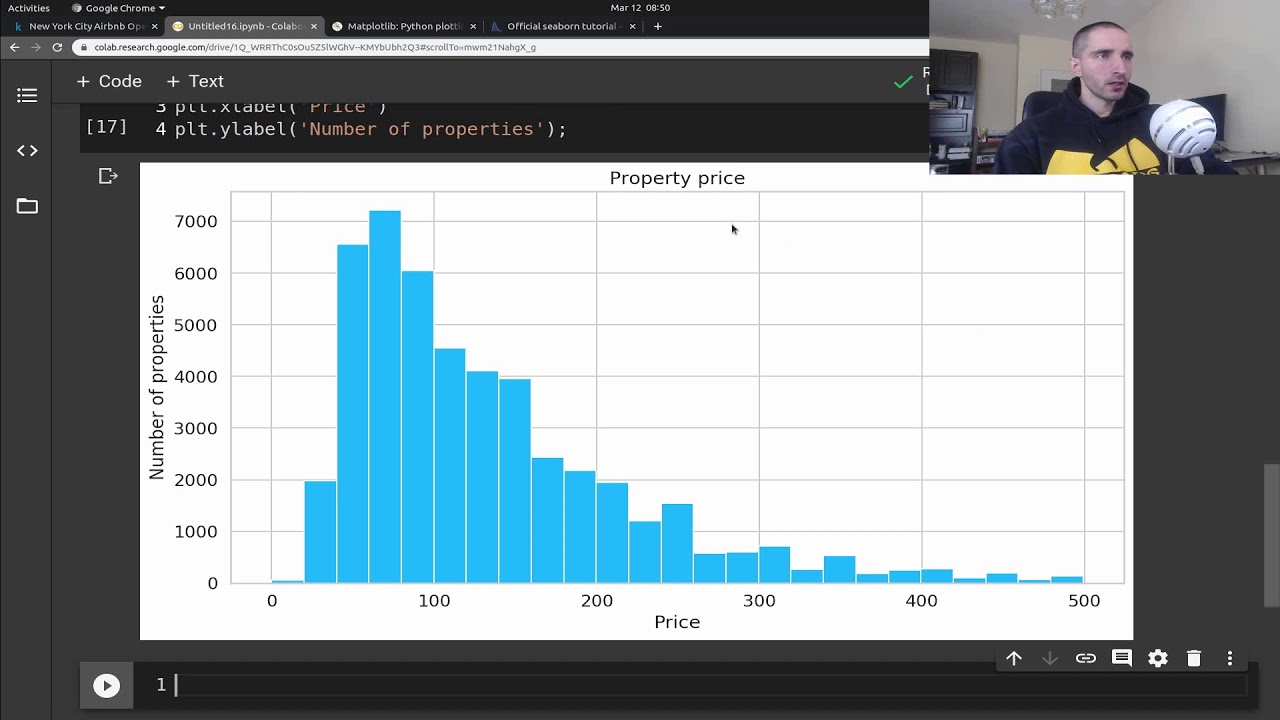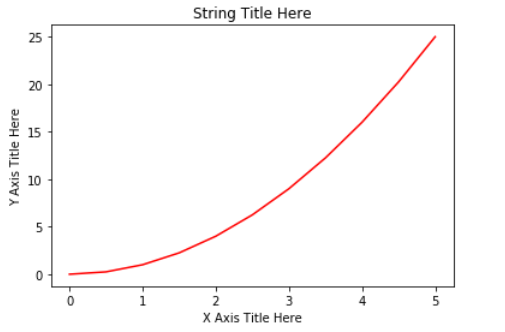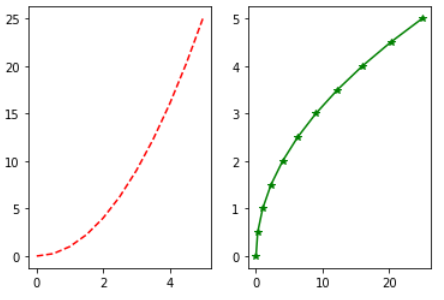1619809560

# Data Visualization Tutorial for Beginners with Matplotlib in Python | Exploratory Data Analysis

Learn how to build an intuition about a Machine Learning problem by creating some fundamental with Matplotlib and Seaborn

⭐️ Tutorial Contents ⭐️

(07:32​) Reading the data
(08:43​) Histogram
(12:35​) Box Plot
(14:28​) Violin Plot
(16:57​) Bar Chart
(19:35​) Line Chart
(21:38​) Scatter plot
(26:45​) Stacker Bar Plot
(30:08​) Showing an image

#python #matplotlib

## Buddha Community1594088160

## Data Visualization With Python: Matplotlib

Data visualization is the graphical representation of data in a graph, chart or other visual formats. It shows relationships of the data with images.

Python offers multiple graphics libraries, with which you can create interactive, live or highly customizable plots with the given data.

To get a little overview here are a few popular plotting libraries:

In this article, we will learn about creating a different type of plots using the Matplotlib library.

Matplotlib is the most popular plotting library for python, which was designed to have a similar feel to MATLAB’s graphical plotting. It gives you control over every aspect of a plot.

Matplotlib allows you to create reproducible figures using a few lines of code. Let’s learn how to use it! I also encourage you to explore: http://matplotlib.org/.

## Installing Matplotlib

Install it with pip or conda at your command line or the terminal with:-

``````pip install matplotlib
OR
conda install matplotlib
``````

To quickly get started with Matplotlib without installing anything on your local machine, check out Google Colab. It provides Jupyter Notebooks hosted on the cloud for free which are associated with your Google Drive account and it comes with all the important packages pre-installed.

## Necessary Imports

`pyplot` is a module of Matplotlib that makes this library work like MATLAB. Import the `matplotlib.pyplot` module under the name `plt` (the tidy way):

``````import matplotlib.pyplot as plt
import numpy as np # for working with arrays
``````

## Making a Simple Plot

We pass two NumPy arrays(x and y) and ‘r’ as arguments to Pyplot’s `plot()` function. Here ‘r’ is for red colour, x elements will appear on x-axis and y elements will appear on the y-axis.

``````import matplotlib.pyplot as plt
import numpy as np

x = np.array([0, 0.5, 1, 1.5, 2, 2.5, 3, 3.5, 4, 4.5, 5])
y = x ** 2 # y is now a list with elements of x to the power 2

plt.plot(x, y, 'r')
plt.xlabel('X Axis Title Here')
plt.ylabel('Y Axis Title Here')
plt.title('String Title Here')
plt.show()
# The plot below is the output of this program.
``````Creating Multiple Plots on The Same Canvas

`subplot()`: a method of pyplot, divides the canvas into `nrows` x `ncols` parts and using `plot_number` argument you can choose the plot.

Syntax: `subplot(nrows, ncols, plot_number)`

In the below example, using `plt.plot(x, y, 'r--’)`we plot a red coloured graph with line style ‘- -’ between x and y at plot_number=1.

``````import matplotlib.pyplot as plt
import numpy as np

x = np.array([0, 0.5, 1, 1.5, 2, 2.5, 3, 3.5, 4, 4.5, 5])
y = x ** 2
plt.subplot(1,2,1) # subplot(nrows, ncols, plot_number)
plt.plot(x, y, 'r--') # r-- meaning colour red with -- pattern
plt.subplot(1,2,2)
plt.plot(y, x, 'g*-') # g*- meaning colour green with *- pattern
# The plot below is the output of this program.
``````For making it more simple `subplots()` method can be used instead of `subplot()`. You will see its example in “Creating Multiple plots on The Same Canvas” under “Matplotlib Object-Oriented Method”.

#data-science #matplotlib #data-visualization #python #plotting-data #data analysis1603270800

## Graphical Approach to Exploratory Data Analysis in Python

Exploratory Data Analysis (EDA) is one of the most important aspect in every data science or data analysis problem. It provides us greater understanding on our data and can possibly unravel hidden insights that aren’t that obvious to us. The first article I’ve wrote on Medium is also on performing EDA in R, you can check it out here. This post will focus more on graphical EDA in Python using matplotlib, regression line and even motion chart!

## Dataset

The dataset we are using for this article can be obtained from Gapminder, and drilling down into _Population, Gender Equality in Education _and Income.

The _Population _data contains yearly data regarding the estimated resident population, grouped by countries around the world between 1800 and 2018.

The Gender Equality in Education data contains yearly data between 1970 and 2015 on the ratio between female to male in schools, among 25 to 34 years old which includes primary, secondary and tertiary education across different countries

The _Income _data contains yearly data of income per person adjusted for differences in purchasing power (in international dollars) across different countries around the world, for the period between 1800 and 2018.

## EDA on Population

Let’s first plot the population data over time, and focus mainly on the three countries Singapore, United States and China. We will use `matplotlib `library to plot 3 different line charts on the same figure.

``````import pandas as pd
import matplotlib.pylab as plt
%matplotlib inline

## read in data
## plot for the 3 countries
plt.plot(population.Year,population.Singapore,label="Singapore")
plt.plot(population.Year,population.China,label="China")
plt.plot(population.Year,population["United States"],label="United States")
## add legends, labels and title
plt.legend(loc='best')
plt.xlabel('Year')
plt.ylabel('Population')
plt.title('Population Growth over time')
plt.show()
``````

#exploratory-data-analysis #data-analysis #data-science #data-visualization #python1617988080

## How To Blend Data in Google Data Studio For Better Data Analysis

Using data to inform decisions is essential to product management, or anything really. And thankfully, we aren’t short of it. Any online application generates an abundance of data and it’s up to us to collect it and then make sense of it.

Google Data Studio helps us understand the meaning behind data, enabling us to build beautiful visualizations and dashboards that transform data into stories. If it wasn’t already, data literacy is as much a fundamental skill as learning to read or write. Or it certainly will be.

Nothing is more powerful than data democracy, where anyone in your organization can regularly make decisions informed with data. As part of enabling this, we need to be able to visualize data in a way that brings it to life and makes it more accessible. I’ve recently been learning how to do this and wanted to share some of the cool ways you can do this in Google Data Studio.

#google-data-studio #blending-data #dashboard #data-visualization #creating-visualizations #how-to-visualize-data #data-analysis #data-visualisation1625001660

## Exploratory Data Analysis in Few Seconds

EDA is a way to understand what the data is all about. It is very important as it helps us to understand the outliers, relationship of features within the data with the help of graphs and plots.

EDA is a time taking process as we need to make visualizations between different features using libraries like Matplot, seaborn, etc.

There is a way to automate this process by a single line of code using the library Pandas Visual Analysis.

## About Pandas Visual Analysis

1. It is an open-source python library used for Exploratory Data Analysis.
2. It creates an interactive user interface to visualize datasets in Jupyter Notebook.
3. Visualizations created can be downloaded as images from the interface itself.
4. It has a selection type that will help to visualize patterns with and without outliers.

## Implementation

1. Installation
2. 2. Importing Dataset
3. 3. EDA using Pandas Visual Analysis

## Understanding Output

Let’s understand the different sections in the user interface :

1. Statistical Analysis: This section will show the statistical properties like Mean, Median, Mode, and Quantiles of all numerical features.
2. Scatter Plot-It shows the Distribution between 2 different features with the help of a scatter plot. you can choose features to be plotted on the X and Y axis from the dropdown.
3. Histogram-It shows the distribution between 2 Different features with the help of a Histogram.

#data-analysis #machine-learning #data-visualization #data-science #data analysis #exploratory data analysis1621635960

## Exploratory Data Analysis in Python: What You Need to Know?

Exploratory Data Analysis (EDA) is a very common and important practice followed by all data scientists. It is the process of looking at tables and tables of data from different angles in order to understand it fully. Gaining a good understanding of data helps us to clean and summarize it, which then brings out the insights and trends which were otherwise unclear.

EDA has no hard-core set of rules which are to be followed like in ‘data analysis’, for example. People who are new to the field always tend to confuse between the two terms, which are mostly similar but different in their purpose. Unlike EDA, data analysis is more inclined towards the implementation of probabilities and statistical methods to reveal facts and relationships among different variants.

Coming back, there is no right or wrong way to perform EDA. It varies from person to person however, there are some major guidelines commonly followed which are listed below.

• Handling missing values: Null values can be seen when all the data may not have been available or recorded during collection.
• Removing duplicate data: It is important to prevent any overfitting or bias created during training the machine learning algorithm using repeated data records
• Handling outliers: Outliers are records that drastically differ from the rest of the data and don’t follow the trend. It can arise due to certain exceptions or inaccuracy during data collection
• Scaling and normalizing: This is only done for numerical data variables. Most of the time the variables greatly differ in their range and scale which makes it difficult to compare them and find correlations.
• Univariate and Bivariate analysis: Univariate analysis is usually done by seeing how one variable is affecting the target variable. Bivariate analysis is carried out between any 2 variables, it can either be numerical or categorical or both.

We will look at how some of these are implemented using a very famous ‘Home Credit Default Risk’ dataset available on Kaggle here. The data contains information about the loan applicant at the time of applying for the loan. It contains two types of scenarios:

• The client with payment difficulties: he/she had late payment more than X days

on at least one of the first Y instalments of the loan in our sample,

• All other cases: All other cases when the payment is paid on time.

We’ll be only working on the application data files for the sake of this article.

#data science #data analysis #data analysis in python #exploratory data analysis in python